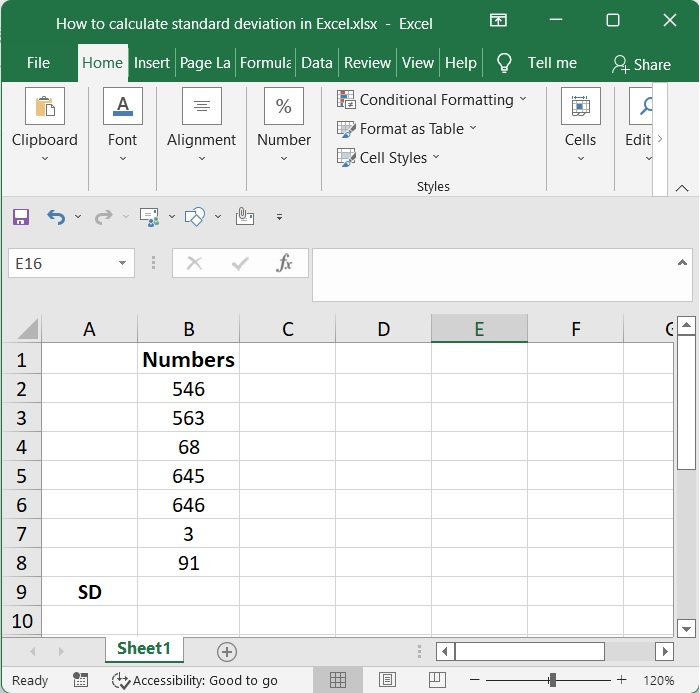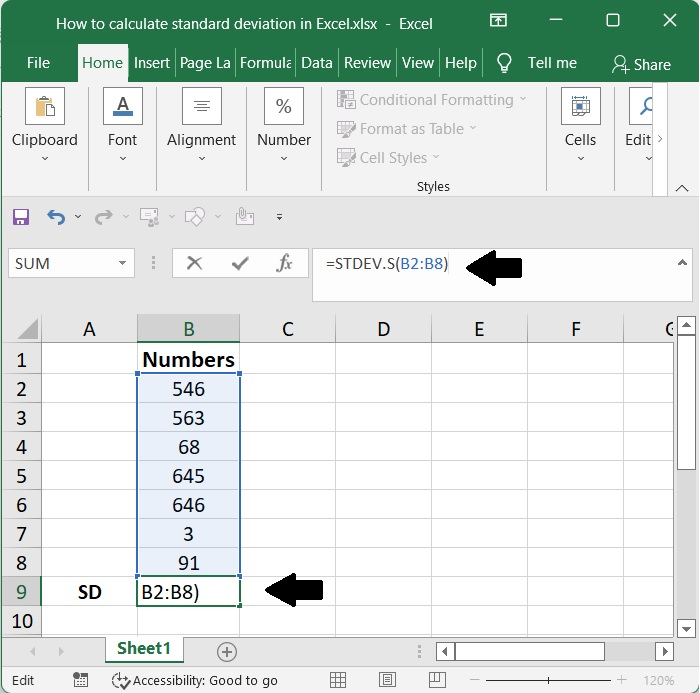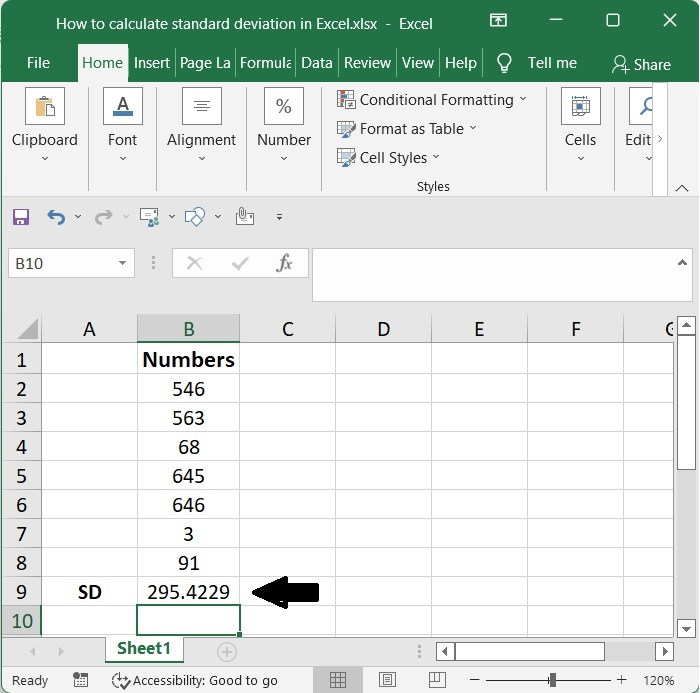# How to calculate standard deviation in Excel?

A number's standard deviation gives you an idea of how much it deviates from the average of all the values in the sample. The fact that each of the numbers is identical to the others indicates that there is no variance. As a direct consequence of this, the numbers have just a standard deviation that is equal to zero.

The STDEV function has been around for a very long time. The new STEDV.S function, which is included in Microsoft Excel and provides the same exact result, is the one that the software company advises using.

We can compute the standard deviation by making use of the STEDV.S function.

STDEV.S(number1,[number2],…)


Here,

• Number 1 is an essential part of the formula and must be included. The first element in the sample of a number, which matches to the first number argument, is the element 1. Alternately, you may use a named range, a singular array, or even a reference to an array in place of the parameters that were previously separated by commas.

• Number2 is an optional parameter. You are allowed to utilise an extra 254 arguments if you so like.

## Step 1

Now that we have some numbers of data, we can compute the standard deviation by making use of the STEDV.S function. This will need that we have certain numbers of data.## Step 2

Applying the following formula to this data set will allow you to compute the standard deviation of the data

=STDEV.S(B2:B8)


Calculate the standard deviation of the numbers B2 through B8 by making use of the STDEV.S function. Following that, you may check your result by pressing the enter key.## Step 3

Here is a screenshot of the standard deviation, (here SD), swing in the bellow plot.## Conclusion

In this tutorial, we used a simple example to demonstrate how you can calculate standard deviation in Excel.